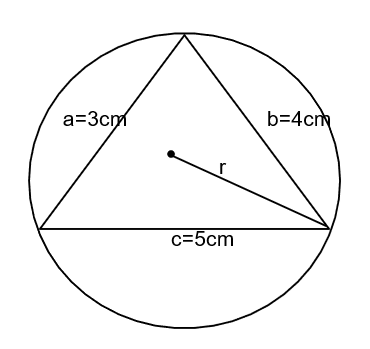Courses
Courses for Kids
Free study material
Offline Centres
MoreLast updated date: 05th Dec 2023
Total views: 282k
Views today: 2.82k

# Calculate the radius of the circumcircle of a triangle, whose lengths are given as $3cm,\,4cm\,and\,5cm$a). $2.1cm$b). $2.2cm$c). $2.3cm$d). $2.5cm$Verified
282k+ views
Hint:Circumscribed circle or circumcircle of a triangle is a circle that passes through all the vertices of triangles. The center of this circle is called the circumcenter of the circle and its radius is called as the circumradius of the circle.
As we know that
$\therefore r = \dfrac{{abc}}{{4\sqrt {s(s - a)(s - b)(s - c)} }}$
Here
s=semi parameter of circle
a, b, and c=sides

Complete step-by-step solution:
Given,$a = 3cm$
$b = 4cm$
$c = 5cm$
Semi perimeter of triangle
As we know that
$\therefore s = \dfrac{{a + b + c}}{2}$
Put the values
$\Rightarrow s = \dfrac{{3 + 4 + 5}}{2}$
Simplify
$\Rightarrow s = 6cm$
Now the radius of circumcenter is
As we know that,
$\therefore r = \dfrac{{abc}}{{4\sqrt {s(s - a)(s - b)(s - c)} }}$
Put the values
$\Rightarrow r = \dfrac{{3 \times 4 \times 5}}{{4\sqrt {6(6 - 3)(6 - 4)(6 - 5)} }}$
Simplify
$\Rightarrow r = \dfrac{{60}}{{4\sqrt {6 \times 3 \times 2 \times 1} }}$
$\Rightarrow r = \dfrac{{60}}{{4 \times 6}}$
$\Rightarrow r = 2.5cm$
The answer is (D) $2.5cm$.

Note: All the vertices of a triangle are equidistant from the circumcenter. In an acute-angled triangle, the circumcenter lies inside the triangle. In an obtuse-angled triangle, it lies outside of the triangle. Circumcenter lies at the midpoint of the hypotenuse side of a right-angled triangle.